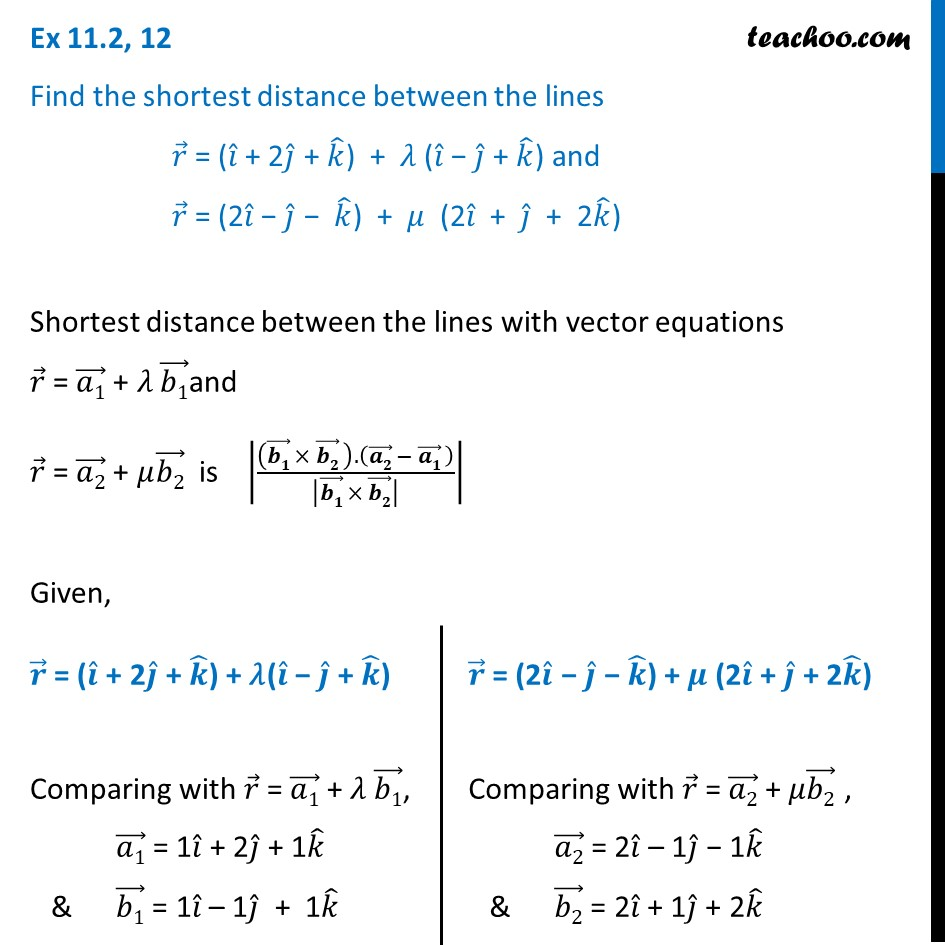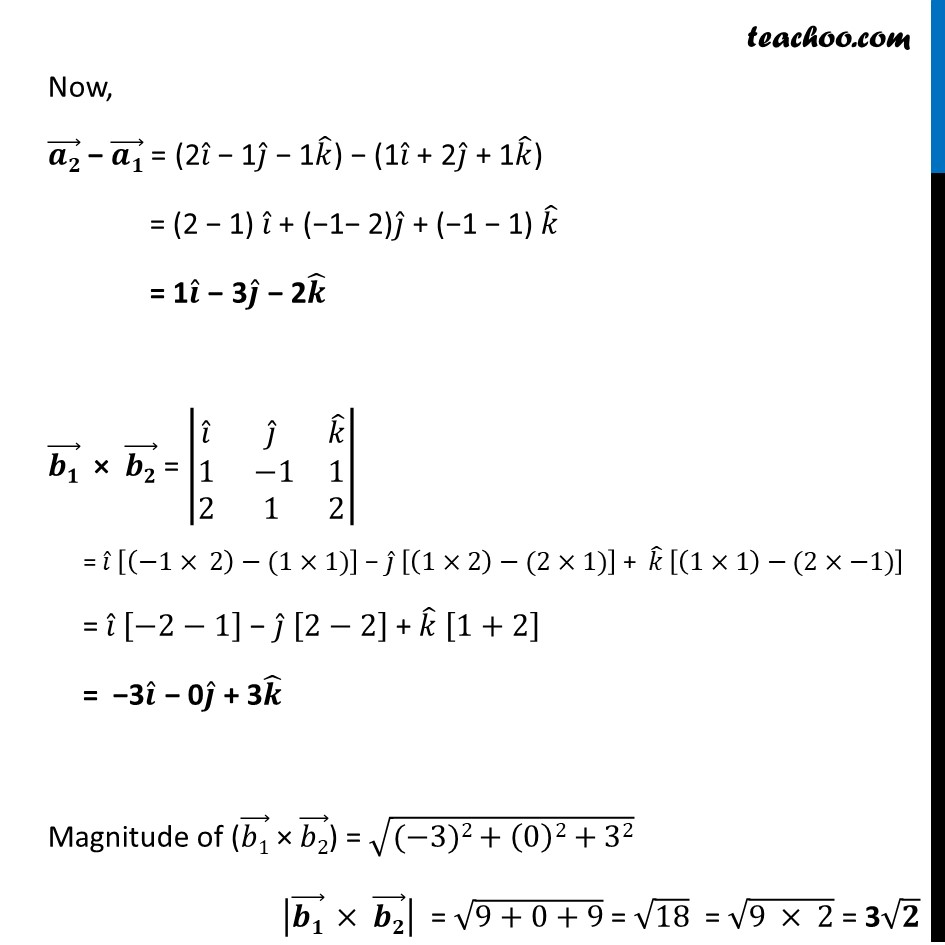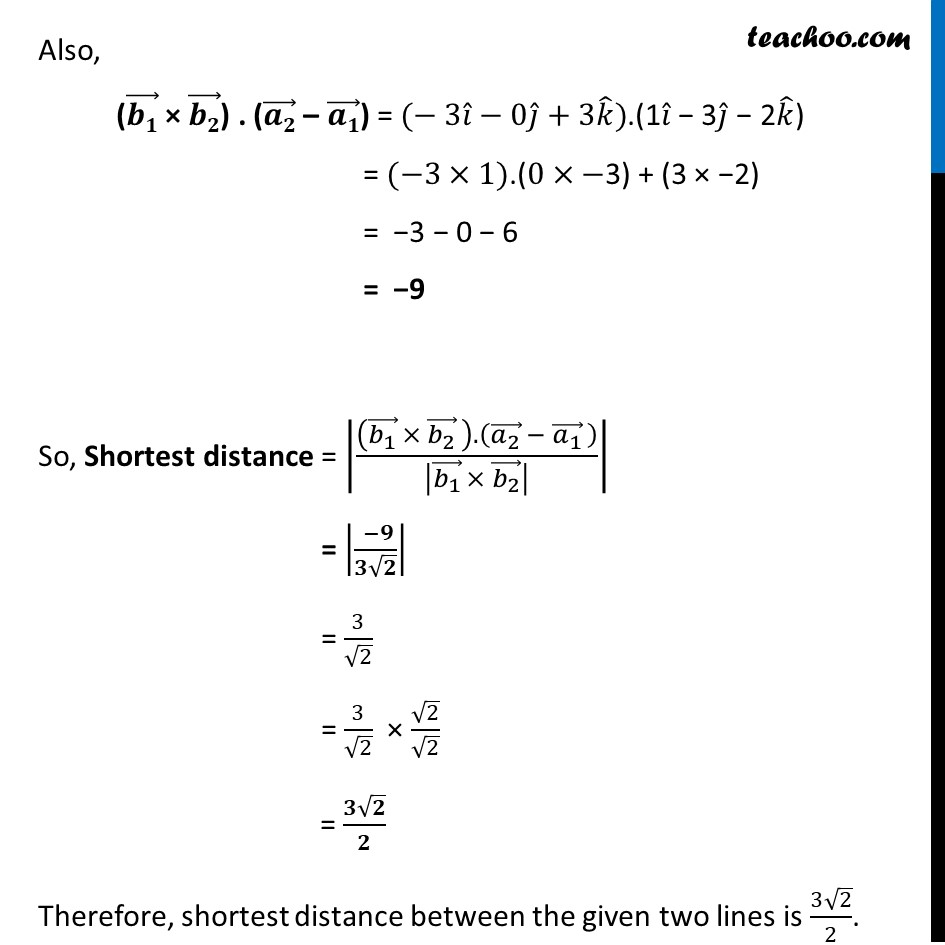Ex 11.2

Chapter 11 Class 12 Three Dimensional Geometry
Serial order wiseLearn in your speed, with individual attention - Teachoo Maths 1-on-1 Class

### Transcript

Ex 11.2, 12 Find the shortest distance between the lines 𝑟 ⃗ = (𝑖 ̂ + 2𝑗 ̂ + 𝑘 ̂) + 𝜆 (𝑖 ̂ − 𝑗 ̂ + 𝑘 ̂) and 𝑟 ⃗ = (2𝑖 ̂ − 𝑗 ̂ − 𝑘 ̂) + 𝜇 (2𝑖 ̂ + 𝑗 ̂ + 2𝑘 ̂) Shortest distance between the lines with vector equations 𝑟 ⃗ = (𝑎1) ⃗ + 𝜆 (𝑏1) ⃗and 𝑟 ⃗ = (𝑎2) ⃗ + 𝜇(𝑏2) ⃗ is |(((𝒃𝟏) ⃗ × (𝒃𝟐) ⃗ ).((𝒂𝟐) ⃗ − (𝒂𝟏) ⃗ ))/|(𝒃𝟏) ⃗ × (𝒃𝟐) ⃗ | | Given, 𝒓 ⃗ = (𝒊 ̂ + 2𝒋 ̂ + 𝒌 ̂) + 𝜆(𝒊 ̂ − 𝒋 ̂ + 𝒌 ̂) Comparing with 𝑟 ⃗ = (𝑎1) ⃗ + 𝜆 (𝑏1) ⃗, (𝑎1) ⃗ = 1𝑖 ̂ + 2𝑗 ̂ + 1𝑘 ̂ & (𝑏1) ⃗ = 1𝑖 ̂ – 1𝑗 ̂ + 1𝑘 ̂ 𝒓 ⃗ = (2𝒊 ̂ − 𝒋 ̂ − 𝒌 ̂) + 𝝁 (2𝒊 ̂ + 𝒋 ̂ + 2𝒌 ̂) Comparing with 𝑟 ⃗ = (𝑎2) ⃗ + 𝜇(𝑏2) ⃗ , (𝑎2) ⃗ = 2𝑖 ̂ – 1𝑗 ̂ − 1𝑘 ̂ & (𝑏2) ⃗ = 2𝑖 ̂ + 1𝑗 ̂ + 2𝑘 ̂ Now, (𝒂𝟐) ⃗ − (𝒂𝟏) ⃗ = (2𝑖 ̂ − 1𝑗 ̂ − 1𝑘 ̂) − (1𝑖 ̂ + 2𝑗 ̂ + 1𝑘 ̂) = (2 − 1) 𝑖 ̂ + (−1− 2)𝑗 ̂ + (−1 − 1) 𝑘 ̂ = 1𝒊 ̂ − 3𝒋 ̂ − 2𝒌 ̂ (𝒃𝟏) ⃗ × (𝒃𝟐) ⃗ = |■8(𝑖 ̂&𝑗 ̂&𝑘 ̂@1& −1&1@2&1&2)| = 𝑖 ̂ [(−1× 2)−(1×1)] − 𝑗 ̂ [(1×2)−(2×1)] + 𝑘 ̂ [(1×1)−(2×−1)] = 𝑖 ̂ [−2−1] − 𝑗 ̂ [2−2] + 𝑘 ̂ [1+2] = −3𝒊 ̂ − 0𝒋 ̂ + 3𝒌 ̂ Magnitude of ((𝑏1) ⃗ × (𝑏2) ⃗) = √((−3)2+(0)2+32) |(𝒃𝟏) ⃗ × (𝒃𝟐) ⃗ | = √(9+0+9) = √18 = √(9 × 2) = 3√𝟐 Also, ((𝒃𝟏) ⃗ × (𝒃𝟐) ⃗) . ((𝒂𝟐) ⃗ – (𝒂𝟏) ⃗) = (− 3𝑖 ̂−0𝑗 ̂+3𝑘 ̂).(1𝑖 ̂ − 3𝑗 ̂ − 2𝑘 ̂) = (−3×1)".(" 0×−"3)" + (3 × −2) = −3 − 0 − 6 = −9 So, Shortest distance = |(((𝑏_1 ) ⃗ × (𝑏_2 ) ⃗ ).((𝑎_2 ) ⃗ − (𝑎_1 ) ⃗ ))/|(𝑏_1 ) ⃗ × (𝑏_2 ) ⃗ | | = |( −𝟗)/(𝟑√𝟐)| = 3/√2 = 3/√2 × √2/√2 = (𝟑√𝟐)/𝟐 Therefore, shortest distance between the given two lines is (3√2)/2.